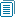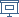FLOC 2018: FEDERATED LOGIC CONFERENCE 2018
LCC ON FRIDAY, JULY 13TH

View: session overviewtalk overviewside by side with other conferences

09:30-10:30 Session 84B: LCC Invited Talk: Mikolaj Bojanczyk
Location: Maths L5
09:30
On polynomial time for infinite structures

ABSTRACT. I will discuss algorithms that process certain kinds of infinite structures (hereditarily definable sets with atoms). An example of such a structure is a directed graph, where the vertices are 10-tuples of rational numbers, and the edge relation is given by a quantifier-free formula that uses the ordering of the rational numbers (but does not use other structure, like addition or multiplication). An example of a decision problem is: given two such graphs decide if they are isomorphic. (For this particular example, it is not known if an algorithm exists.) I will be concerned with two questions: (a) which problems should be called “decidable”; and (b) which problems should be called “polynomial time”.

(Joint work with Szymon Toruńczyk)

10:30-11:00Coffee Break
11:00-12:30 Session 86F: LCC: Contributed Talks
Location: Maths L5
11:00
Traversal-invariant definability and Logarithmic-space computationABSTRACT. In the presence of arithmetic, order-invariant definability in first-order logic captures constant parallel time, i.e. uniform AC⁰ [I]. The ordering is necessary to replicate the presentation of a structure on the input tape of a machine. What if that ordering was in fact a traversal of the structure? We show that an analogous notion of traversal-invariant definability characterizes deterministic logarithmic space (L) and that breadth-first traversals characterize nondeterministic logarithmic space (NL). The surprising feature of these characterizations is that we can work entirely within the framework of first-order logic without any extensions, other than the traversals themselves.

11:15
A recursion-theoretic characterisation of the positive polynomial-time functions
SPEAKER: Anupam Das

ABSTRACT. We extend work of Lautemann, Schwentick and Stewart '96 on characterisations of the 'positive' polynomial-time predicates (posP, also called mP by Grigni and Sipser '92) to function classes. Our main result is the obtention of a function algebra for the positive polynomial-time functions (posFP), by imposing a simple uniformity condition on the bounded recursion operator in Cobham's characterisation of FP.

11:30
Feasibly constructive proofs of succinct weak circuit lower boundsSPEAKER: Ján Pich

ABSTRACT. We ask for feasibly constructive proofs of known circuit lower bounds for explicit functions on bit strings of length n. In 1995 Razborov showed that many can be proved in Cook's theory PV, a bounded arithmetic formalizing polynomial time reasoning. He formalized circuit lower bound statements for small n of doubly logarithmic order. A more common formalization, considered in Krajíček's 1995 textbook, assumes n only of logarithmic order. It is open whether PV proves known lower bounds in such succinct formalizations. We give such proofs in Jeřábek's theory of approximate counting APC_1, an extension of PV formalizing probabilistic polynomial time reasoning. Specifically, we prove in APC_1 lower bounds for the parity function and AC^0, for the mod q counting function and AC^0[p] (for some n of intermediate order), and for the k-clique function and monotone circuits. We also formalize Razborov and Rudich's natural proof barrier. Further, we ask for feasibly constructible propositional proofs of circuit lower bounds. We discuss two ways to succinctly express circuit lower bounds by propositional formulas of polynomial size n^O(1) or at least much smaller than size 2^O(n) of the common formula based on the truth table of the function: one via feasible functions witnessing errors of circuits trying to compute some hard function, and one via the anticheckers of Lipton and Young 1994 or partial truth tables. Our APC_1 formalizations can be applied to derive a conditional upper bound on succinct propositional formulas obtained by witnessing extracted from the APC_1 proofs. Namely, we show these formulas have short Extended Frege EF proofs from general circuit lower bounds expressed by the common``truth-table" formulas. We also show how to construct in quasipolynomial time propositional proofs of quasipolynomial size tautologies expressing AC^0[p] quasipolynomial size lower bounds; these proofs are in Jeřábek's proof system WF. The last result is proved by formalizing a variant of Razborov's and Rudich's naturalization of Smolensky's proof for partial functions on the propositional level.

11:45
Computability over locally finite structures from algebra

ABSTRACT. Working with a particular notion of computability over general, first- order structures, we argue that computability classes over certain locally finite structures should capture Turing machine complexity classes. We exhibit this phenomenon for some locally finite structures from algebra.

12:00
A General Equational Framework for Static Profiling of Parametric Resource UsageABSTRACT. For some applications, standard resource analyses do not provide the information required. Such analyses estimate the total resource usage of a program (without executing it) as functions on input data sizes. However, some applications require knowing how such total resource usage is distributed over selected parts of a program. We propose a novel, general, and flexible framework for setting up cost equations/relations which can be instantiated for performing a wide range of resource usage analyses, including both static profiling and the inference of the standard notion of cost. We extend and generalize standard resource analysis techniques, so that the relations generated include additional Boolean control variables for switching on or off different terms in the relations, as required by the desired resource usage profile. We also instantiate our framework to perform static profiling of accumulated cost (also parameterized by input data sizes). Such information is much more useful to the software developer than the standard notion of cost: it identifies the parts of the program that have the greatest impact on the total program cost, and which therefore should be optimized first. We also report on an implementation of our framework within the CiaoPP system, and its instantiation for accumulated cost, and provide some experimental results. In addition to generality, our new method brings important advantages over our previous approach based on a program transformation, including support for non-deterministic programs, better and easier integration in the compiler, and higher efficiency.

12:15
Completeness in the Second Level of the Polynomial Time Hierarchy through Syntactic PropertiesABSTRACT. In the mid-90's Neil Immerman and José Medina initiated the search for syntactic tools to prove NP-completeness. They conjectured that if a problem defined by the conjunction of an Existential Second Order sentence and a First Order Formula is NP-complete then the Existential Second Order formula denes an NP-complete problem. This property was called superfluity of First Order Logic with respect to NP. Few years later it was proved by Nerio Borges and Blai Bonet that superfluity is true for the universal fragment of First Order Logic and with respect not only to NP but for a major rank of complexity classes. Our work extends that result to the Second Level of the Polynomial-Time Hierarchy

12:30-14:00Lunch Break
14:00-15:30 Session 87F: LCC: Martin Hofmann Memorial Session
Location: Maths L5
14:00
Characterizing Complexity Classes in Presence of Higher-Order Functions — How Martin Hofmann Contributed to Shaping ICC

ABSTRACT. Implicit computational complexity is concerned with characterizing complexity classes with tools coming from logic (e.g. proof theory,  recursion theory) or from programming language theory (e.g. type systems). The presence of higher-order functions makes the task quite hard, given the strong expressive power higher-order functions provide. A key insight is that the expressive power is not provided by the mere *presence* of higher-order functions, but by the possibility of *copying* them. As a consequence, linearity turned out to be a powerful and versatile tool. I will give a survey of some of the results obtained along these lines, starting in the early nineties until today. This talk is dedicated to the memory of Martin Hofmann: he contributed intensely and decisively to this research line, and he introduced it to me gently but thoroughly.

15:00
Static Prediction of Heap Space Usage for First-Order Functional Programs -- A Retrospective on my First Paper with Martin Hofmann15:30-16:00Coffee Break
16:00-18:00 Session 88D: LCC: Martin Hofmann Memorial Session
Location: Maths L5
16:00
My Journey through Observational Equivalence Research with Martin Hofmann16:30
Memories of Martin
19:00-21:30 Workshops dinner at Keble College

Workshops dinner at Keble College. Drinks reception from 7pm, to be seated by 7:30 (pre-booking via FLoC registration system required; guests welcome).

Location: Keble College